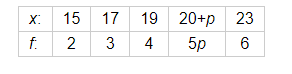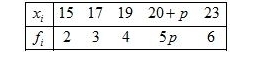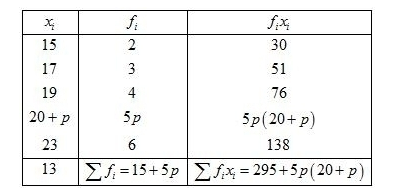# Find the value of p, if the mean of the following distribution is 20.

Question:

Find the value of p, if the mean of the following distribution is 20.Solution:

Given:Mean $=20$

First of all prepare the frequency table in such a way that its first column consist of the values of the variate $\left(x_{i}\right)$ and the second column the corresponding frequencies $\left(f_{i}\right)$.

Thereafter multiply the frequency of each row with corresponding values of variable to obtain third column containing $\left(f_{i} x_{i}\right)$.

Then, sum of all entries in the column second and denoted by $\sum f_{i}$ and in the third column to obtain $\sum f_{i} x_{i}$.We know that mean, $\bar{X}=\frac{\sum f_{i} x_{i}}{\sum f_{i}}$

$20=\frac{295+5 p(20+p)}{15+5 p}$

By using cross multiplication method,

$5 p^{2}+100 p+295=300+100 p$

$\Rightarrow 5 p^{2}=300-295=5$

$\Rightarrow p^{2}=1$

$\Rightarrow p=1$

Hence, $p=1$